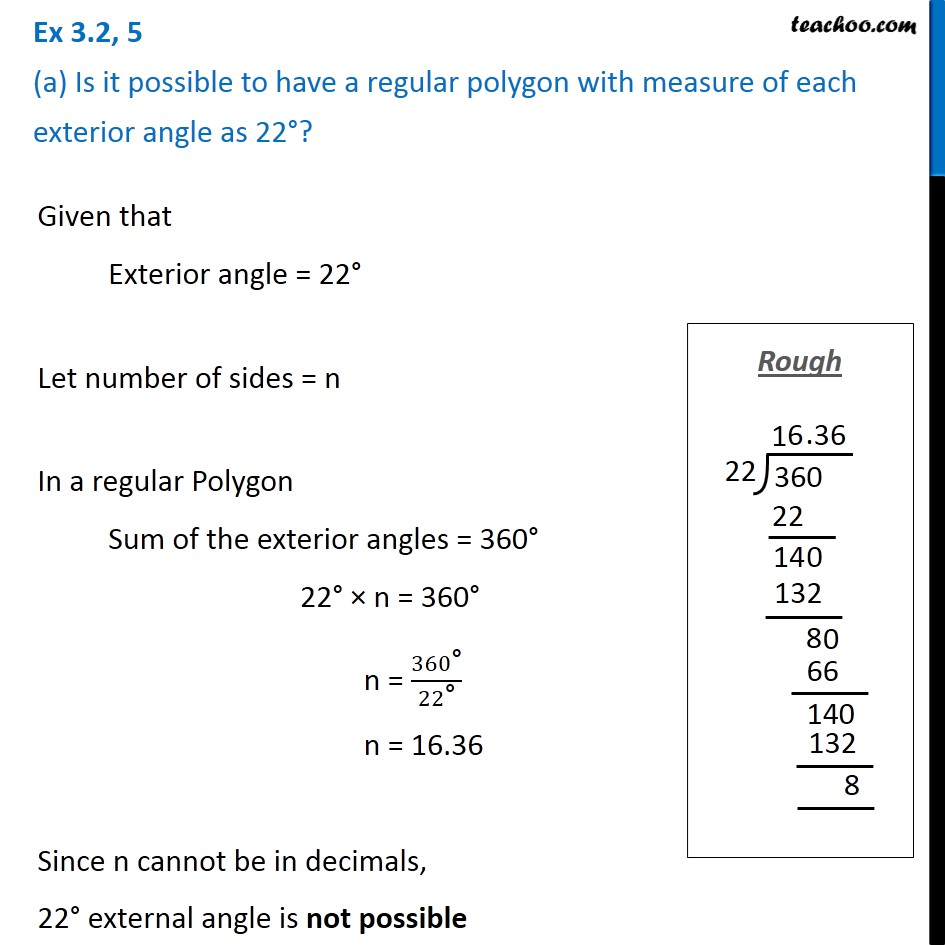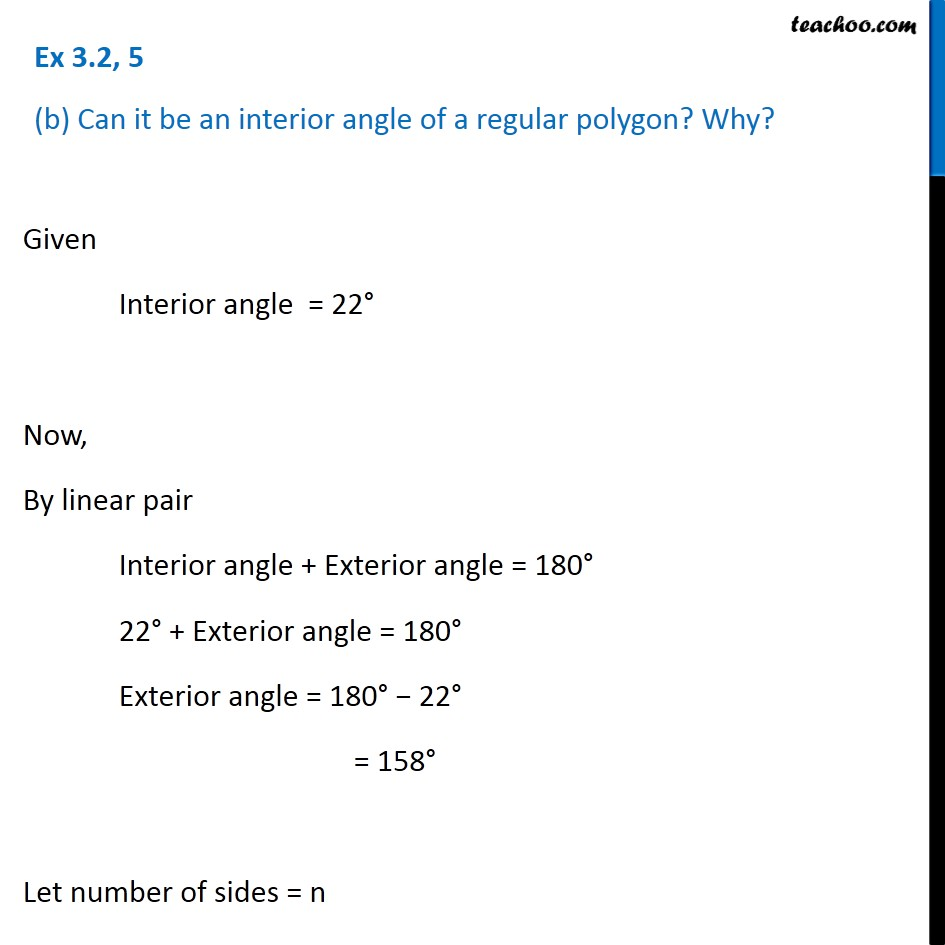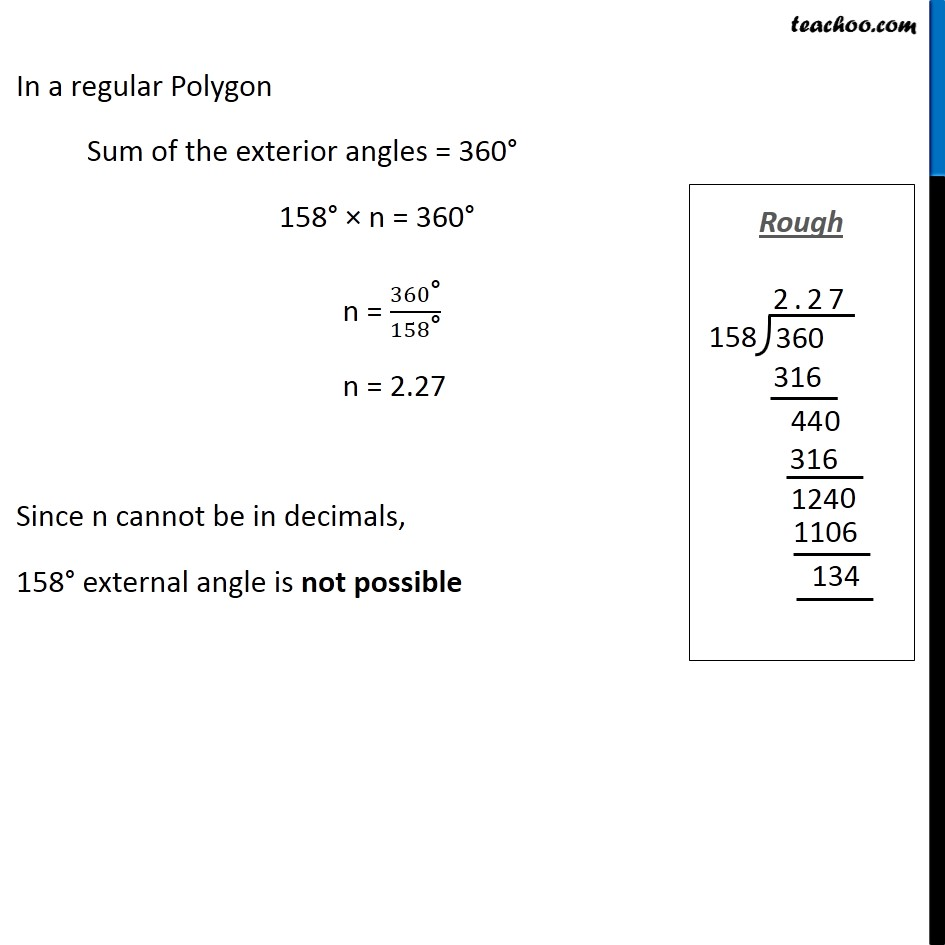Subscribe to our Youtube Channel - https://you.tube/teachoo

1. Chapter 3 Class 8 Understanding Quadrilaterals
2. Concept wise
3. Sum of Exterior Angles of Polygons

Transcript

Ex 3.2, 5 (a) Is it possible to have a regular polygon with measure of each exterior angle as 22°?Given that Exterior angle = 22° Let number of sides = n In a regular Polygon Sum of the exterior angles = 360° 22° × n = 360° n = 360"°" /22"°" n = 16.36 Since n cannot be in decimals, 22° external angle is not possible Ex 3.2, 5 (b) Can it be an interior angle of a regular polygon? Why? Given Interior angle = 22° Now, By linear pair Interior angle + Exterior angle = 180° 22° + Exterior angle = 180° Exterior angle = 180° − 22° = 158° Let number of sides = n In a regular Polygon Sum of the exterior angles = 360° 158° × n = 360° n = 360"°" /158"°" n = 2.27 Since n cannot be in decimals, 158° external angle is not possible

Sum of Exterior Angles of Polygons